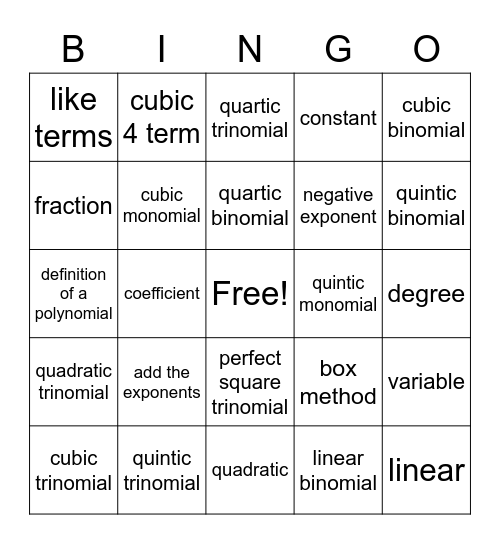# Polynomials BingoThis bingo card has a free space and 34 words: linear monomial, linear binomial, quadratic monomial, quadratic binomial, quadratic trinomial, cubic monomial, cubic binomial, cubic trinomial, cubic 4 term, quartic monomial, quartic binomial, quartic trinomial, quartic 4 term, quartic 5 term, quintic monomial, quintic binomial, quintic trinomial, definition of a polynomial, add the exponents, box method, perfect square trinomial, difference of squares, like terms, degree, quadratic, linear, constant, quotient, negative exponent, fraction, product, coefficient, variable and quantity.

## Play Online